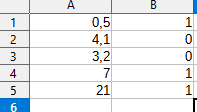# Counting values next to a bool cell

Let’s take a table as an examplehow would I go about summing up values from column A that are in the same row as 1’s in the B column.
All values in column B are boolean.

You have many possibilities:

``````=SUMIF(B1:B30;1;A1:A30)
=SUMIFS(A1:A30;B1:B30;1)
=SUMPRODUCT(A1:A30;B1:B30)
{=SUM(IF(B1:B30;A1:A30;0))}
``````

Pay attention to the curly braces around the last formula - this is an array formula, the input must end with Ctrl+Shift+Enter